# Where should you declare aesthetics? Globally, or geom-by-geom?

Where should you declare aesthetics? Globally or in the geom_*() function? The answer to this question, in some sense is personal preference, because there are simply different ways to get the same job done in the ggplot architecture. My preference is declaring all aesthetic mappings as global unless there are conflicts.

Below is an example that, I hope, will persuade you to my preference. We graph the increase in life expectancy by year for three countries. First, subset some data from the gapminder dataset and we create a scatterplot.

``````library(tidyverse)
df <- gapminder::gapminder %>%
filter(country %in% c("Germany", "United States", "Italy"))

ggplot(df) +
aes(x = year,
y = lifeExp) +
geom_point() ``````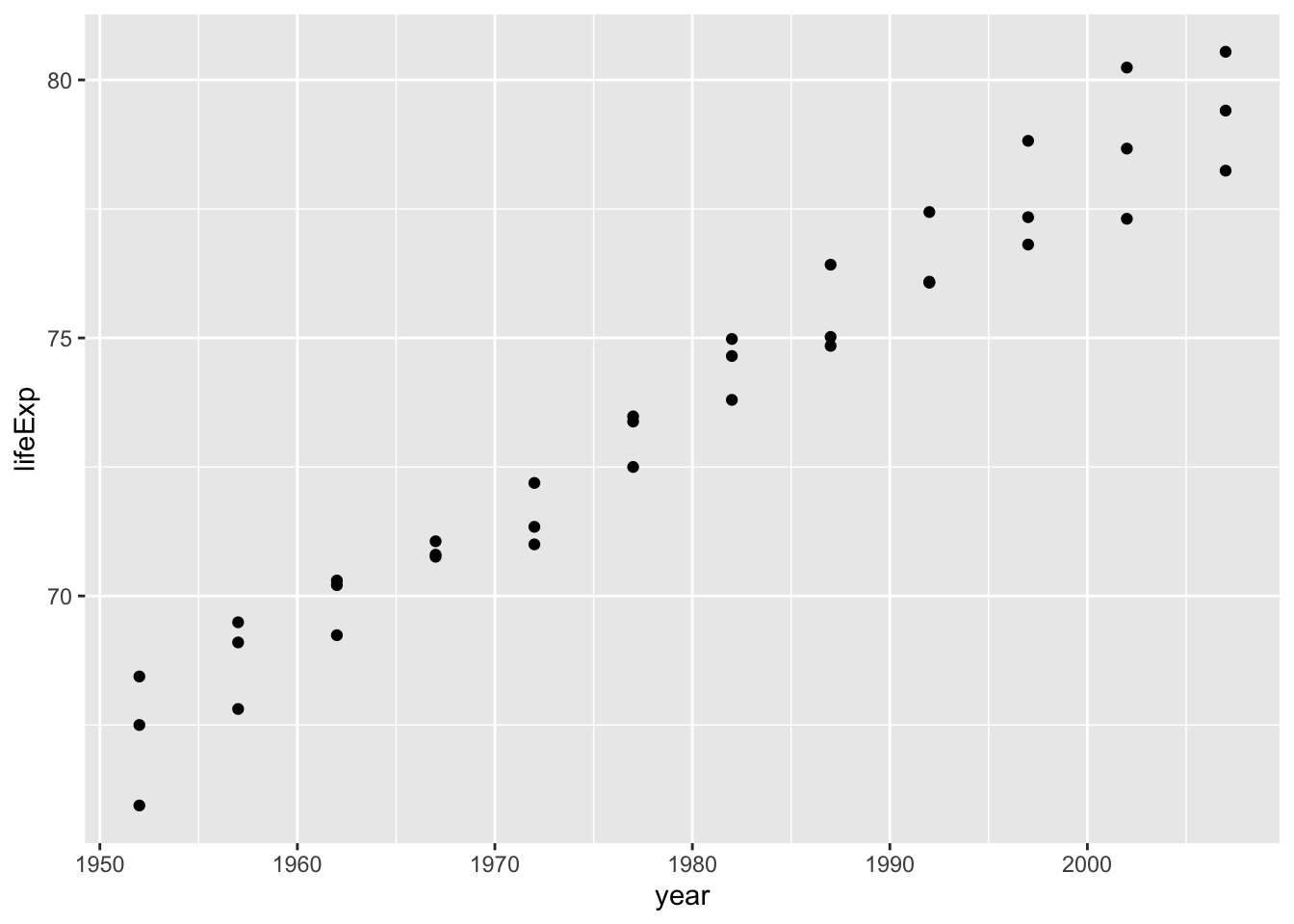Then we might want to add a line connecting the three countries data year by year. We add geom_line, but notice that grouping needs to be declared to distinguish the countries:

``````ggplot(df) +
aes(x = year,
y = lifeExp) +
geom_point() +
geom_line()``````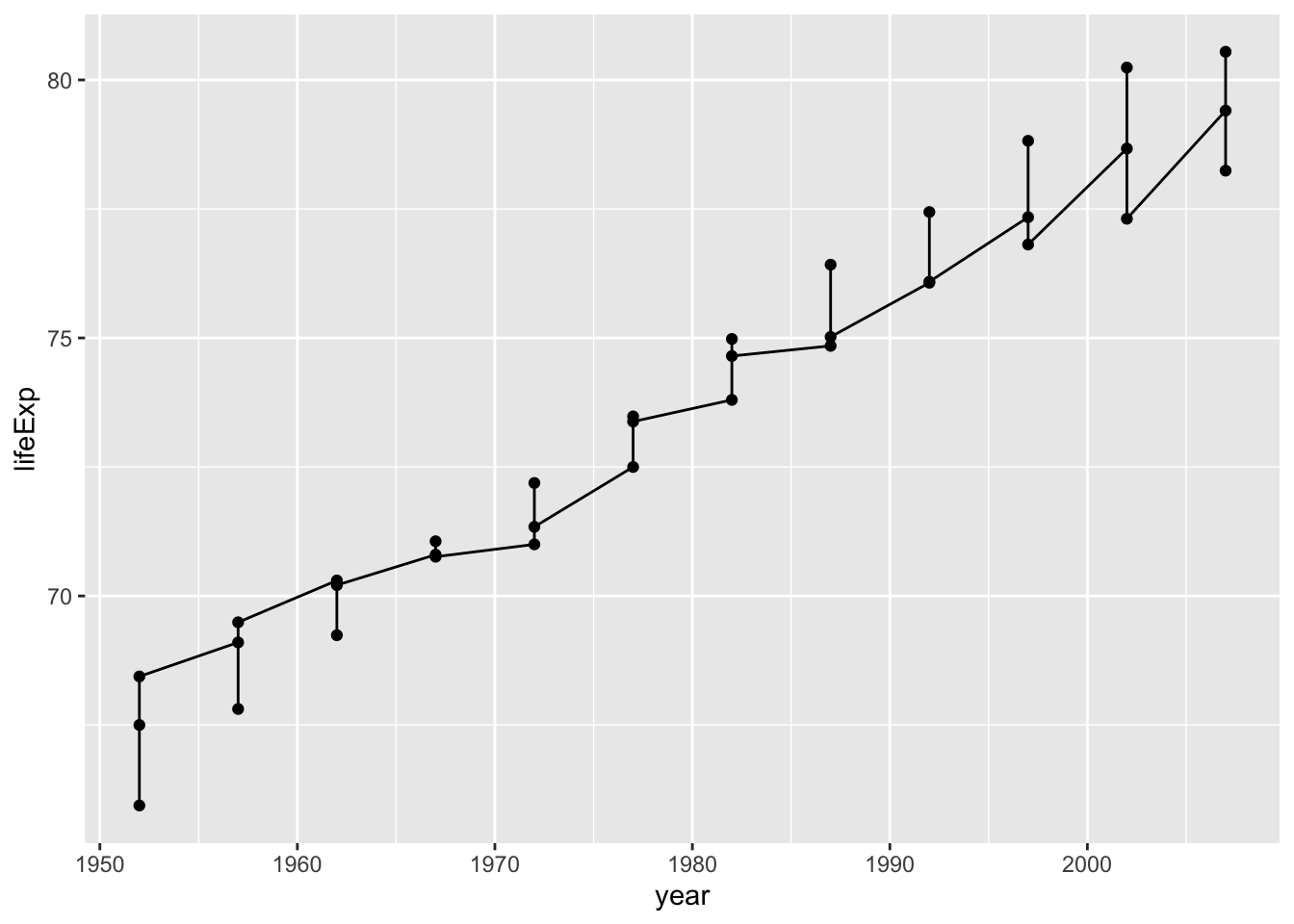Using the grouping, (notice here I’m declaring the grouping as a global aesthetic mapping), the plot makes a bit more sense.

``````ggplot(df) +
aes(x = year,
y = lifeExp,
group = country) +
geom_point() +
geom_line()``````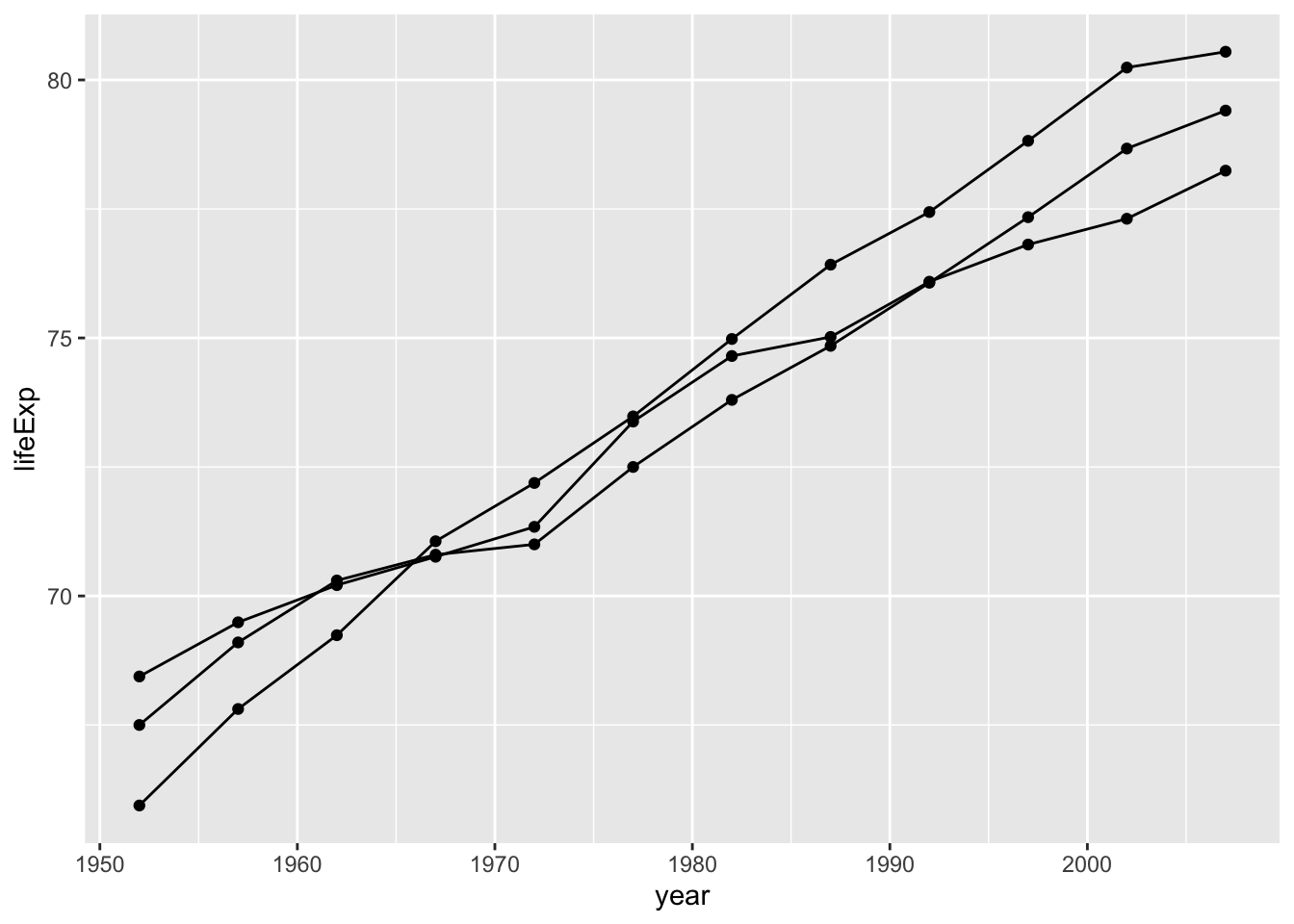Then, given this declaration, I can add a linear trend line with geom_smooth() for each country.

``````ggplot(df) +
aes(x = year,
y = lifeExp,
group = country) +
geom_point() +
geom_line() +
geom_smooth(method = lm)``````
``## `geom_smooth()` using formula 'y ~ x'``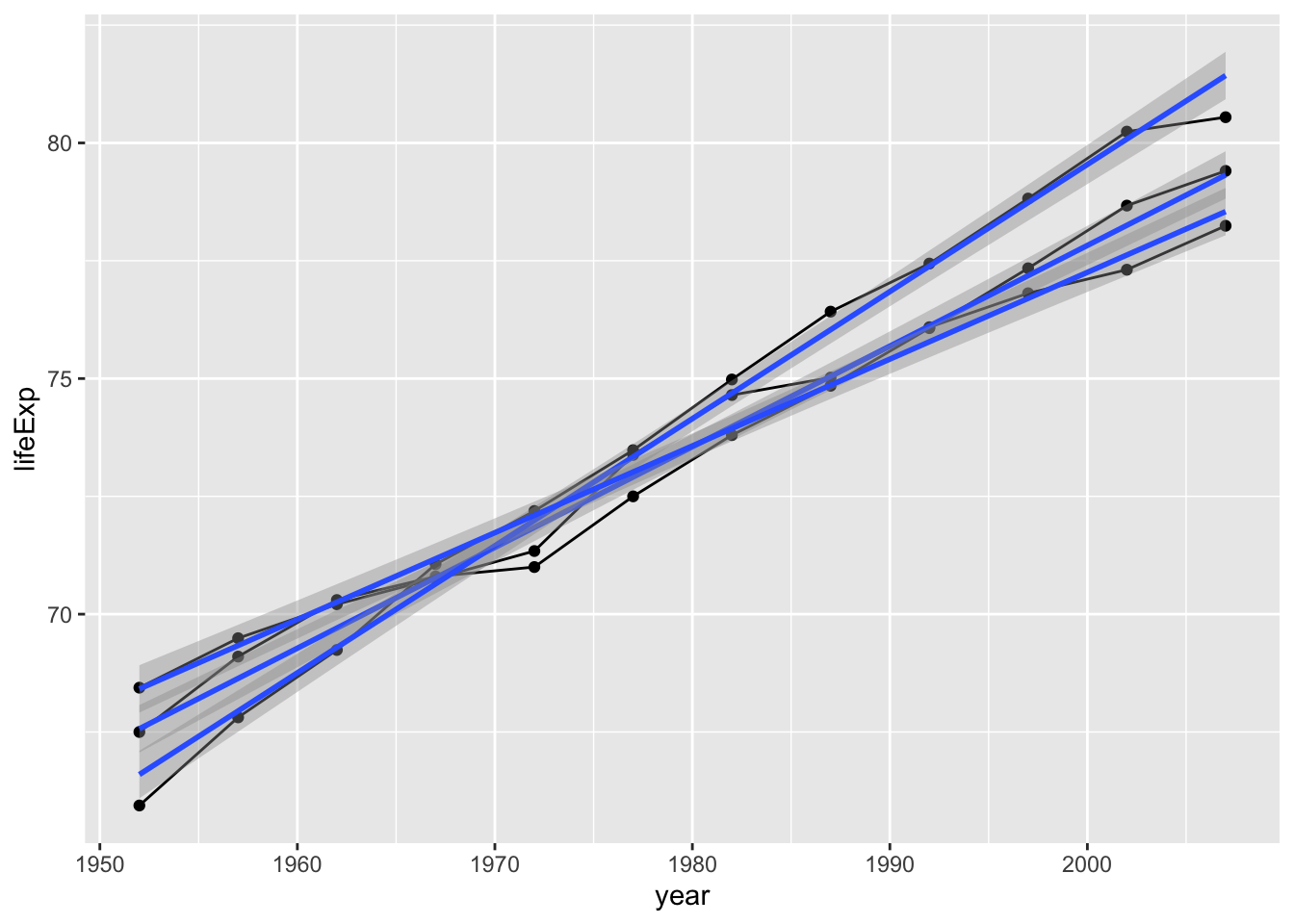This is where I think the gain is. You don’t have to make the grouping declaration twice. (And you can override the global grouping aesthetic, should you so desire, in the geom_smooth() The alternative is redundant:

``````ggplot(df) +
aes(x = year,
y = lifeExp) +
geom_point() +
geom_line(aes(group = country)) +
geom_smooth(aes(group = country), method = lm)``````
``## `geom_smooth()` using formula 'y ~ x'``In conclusion, trust your aesthetic mapping decisions, and just make them global. You might want to use them across more geoms as you build up your plot, and probably you will want consistent aesthetic mapping choices across your geoms. When a conflict arises, then you can simply override the aesthetic as you need to within the specific geom function, as done below.

``````ggplot(df) +
aes(x = year,
y = lifeExp,
group = country) +
geom_point() +
geom_line() +
geom_smooth(aes(group = NULL), method = lm)``````
``## `geom_smooth()` using formula 'y ~ x'``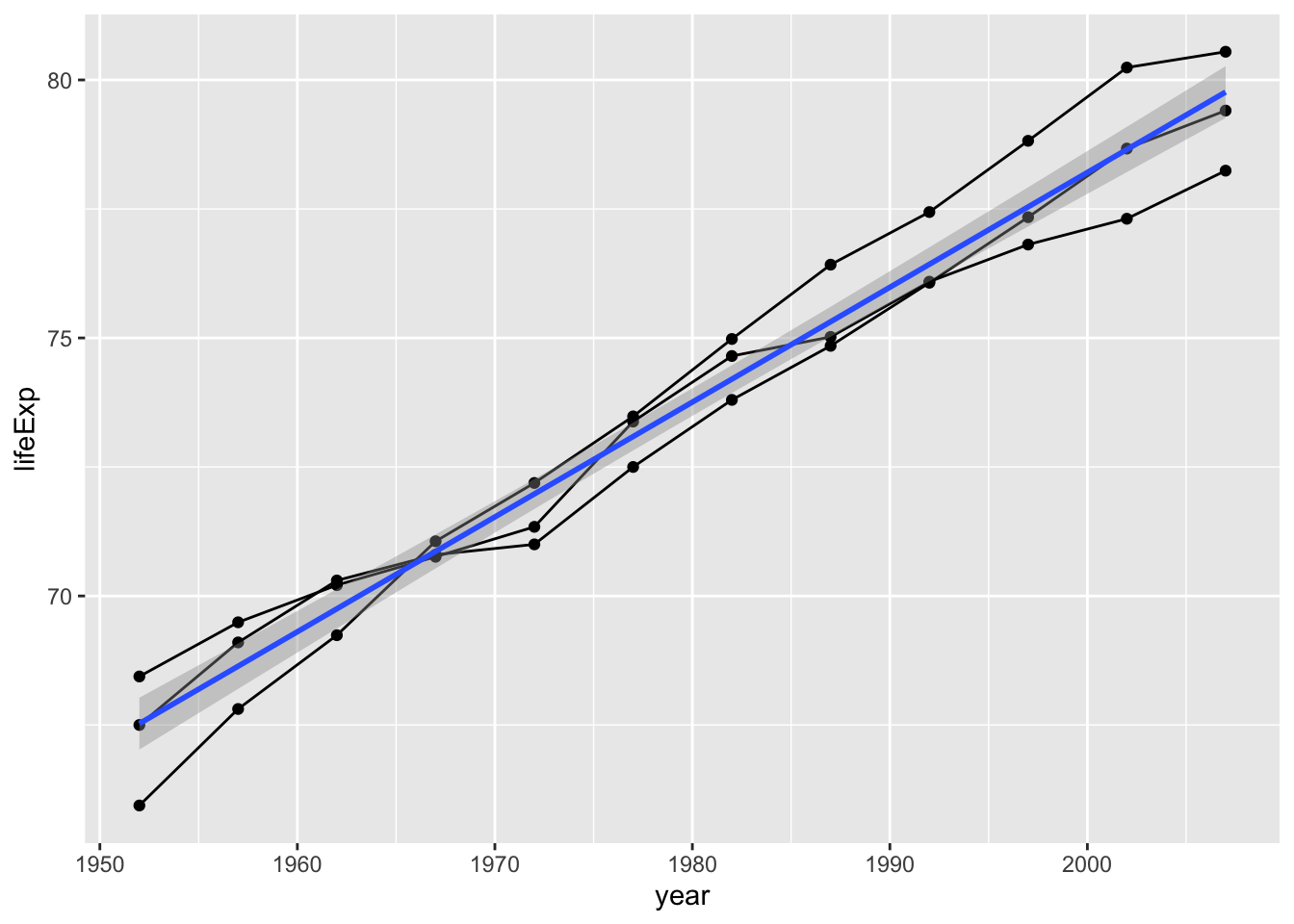##### Evangeline Reynolds
###### Visiting Teaching Assistant Professor

My research interests include international institutions, causal inference, data visualization, and computational social science and pedagogy.